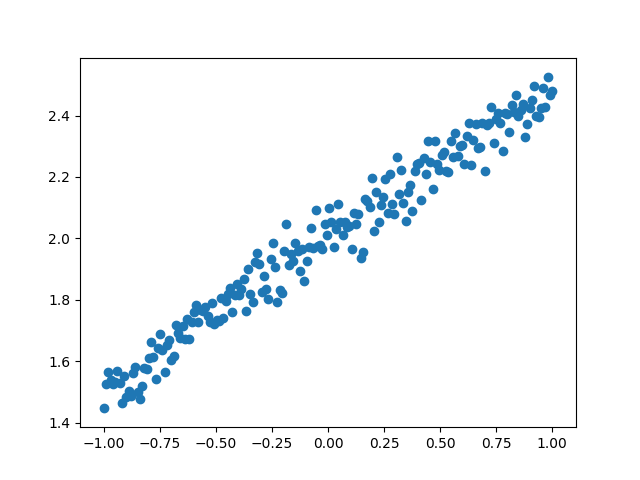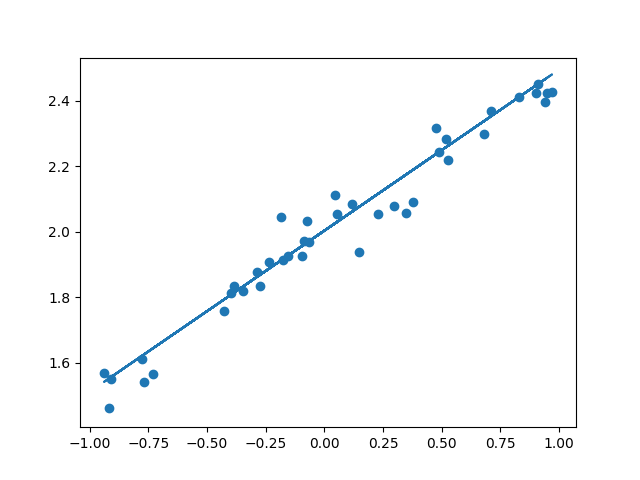1.匯入的模組

`model.add(Dense(64, input_dim=64, W_regularizer=l2(0.01), activity_regularizer=activity_l2(0.01)))`
Dense層包括output_dim（輸出維度）、input_dim（輸入維度）、activation（啟用函式）、weights（權值）、W_regularizer（施加在權值上的正則項）、b_regularizer（施加在偏置向量上的正則項）、activity_regularizer（施加在輸出上的正則項）、W_constraints（施加在權值上的約束項）、b_constraints、bias（布林值，是否包含偏置向量）這些引數。

2.資料的生成

3.建立模型

4.編譯模型

model.compile()來編譯和啟用模型，這裡我們用的損失函式是mse均方誤差；優化器用的是sgd隨機梯度下降。

``compile(self, optimizer, loss, metrics=[], loss_weights=None, sample_weight_mode=None)``

optimizer：優化器，為預定義優化器名或優化器物件，參考優化器
loss：目標函式，為預定義損失函式名或一個目標函式，參考目標函式
metrics：列表，包含評估模型在訓練和測試時的效能的指標，典型用法是 metrics=[‘accuracy’] 如果
Keras中文文件

{‘ouput_a’: ‘accuracy’}
sample_weight_mode：如果你需要按時間步為樣本賦權（2D權矩陣），將該值設為“temporal”。

kwargs：使用TensorFlow作為後端請忽略該引數，若使用Theano作為後端，kwargs的值將會傳遞

5.訓練模型

``train_on_batch(self, x, y, class_weight=None, sample_weight=None)``

6.驗證模型

``evaluate(self, x, y, batch_size=32, verbose=1, sample_weight=None)``

x：輸入資料，與 fit 一樣，是numpy array或numpy array的list
y：標籤，numpy array
batch_size：整數，含義同 fit 的同名引數
verbose：含義同 fit 的同名引數，但只能取0或1
sample_weight：numpy array，含義同 fit 的同名引數

``````import numpy as np
np.random.seed(1337)
from keras.models import Sequential
from keras.layers import Dense
import matplotlib.pyplot as plt
/*建立資料集*/
X=np.linspace(-1,1,200)
np.random.shuffle(X)
Y=0.5*X+2+np.random.normal(0,0.05,(200,))
/*視覺化*/
plt.scatter(X,Y)
plt.show()
X_train,Y_train=X[:160],Y[:160]
X_test,Y_test=X[160:],Y[160:]

/*建立神經網路模型*/
model=Sequential()

/*編譯，選定loss函式和優化器*/
model.compile(loss='mse',optimizer='sgd')

/*訓練過程*/
print('Training--------------')
for  step in range(501):
cost=model.train_on_batch(X_train,Y_train)
if step%100==0:
print('train_cost: ',cost)
/*測試過程*/
print('\nTesting--------------')
cost=model.evaluate(X_test,Y_test,batch_size=40)
print('test cost:',cost)
W, b=model.layers.get_weights()
print('weight= ',W,'\nbiases=',b)

/*結果的視覺化*/
Y_pred=model.predict(X_test)
plt.scatter(X_test,Y_test)
plt.plot(X_test,Y_pred)
plt.show()``````